# Greek number systems

There were no single Greek national standards in the first millennium BC. since the various island states prided themselves on their independence. This meant that they each had their own currency, weights and measures etc. These in turn led to small differences in the number system between different states since a major function of a number system in ancient times was to handle business transactions. However we will not go into sufficient detail in this article to examine the small differences between the system in separate states but rather we will look at its general structure. We should say immediately that the ancient Greeks had different systems for cardinal numbers and ordinal numbers so we must look carefully at what we mean by Greek number systems. Also we shall look briefly at some systems proposed by various Greek mathematicians but not widely adopted.

The first Greek number system we examine is their acrophonic system which was use in the first millennium BC. 'Acrophonic' means that the symbols for the numerals come from the first letter of the number name, so the symbol has come from an abreviation of the word which is used for the number. Here are the symbols for the numbers 5, 10, 100, 1000, 10000.Acrophonic 5, 10, 100, 1000, 10000.

We have omitted the symbol for 'one', a simple '|', which was an obvious notation not coming from the initial letter of a number. For 5, 10, 100, 1000, 10000 there will be only one puzzle for the reader and that is the symbol for 5 which should by P if it was the first letter of Pente. However this is simply a consequence of changes to the Greek alphabet after the numerals coming from these letters had been fixed. By that time the symbols were probably not thought of as coming from the letters so there was no move to change them with changes to the symbols for the letters. The original form of π was G and so Pente was originally Gente.

Now the system was based on the additive principle in a similar way to Roman numerals. This means that 8 is simply V|||, the symbol for five followed by three symbols for one. Here is 1-10 in Greek acrophonic numbers.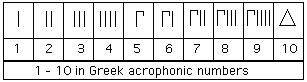1-10 in Greek acrophonic numbers.

If base 10 is used with an additive system without intermediate symbols then many characters are required to express certain numbers. The number 9999 would require 36 symbols in such a system and this is very cumbersome. We have already seen that that Greek acrophonic numbers had a special symbol for 5. This is not surprising for it cuts down the characters required and also presumably arises from counting on fingers. We have 10 fingers but there is 5 on each hand. What is slightly more surprising is that the system had intermediate symbols for 50, 500, 5000, and 50000 but they were not new characters, rather they were composite symbols made from 5 and the symbols for 10, 100, 1000, 10000 respectively. Here is how the composites were formed.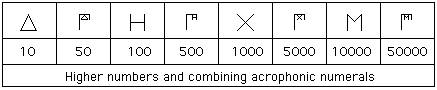Combining acrophonic numerals.

Notice that since there was no positional aspect of the system, there was no need for zero as an empty place holder. The symbol H represented 100 as no problem is created in the representation by the number having no tens or units.

Now this is not the only way in which such composite symbols were created. We have already mentioned that different states used variants of the number system and, although we are not going to examine these in detail, let us at least give some indication by showing some forms of 50 that have been found. Most of these forms are older than the main form of the numerals we have considered being more typical of the period 1500 BC to 1000 BC.Different forms of 50 in different Greek States.

The next point worth noting is that this number system did not really consist of abstract numbers in the way we think of numbers today. Today the number 2 is applied to any collection of two objects and 2 is thought of as an abstract property that all such collections of two objects have in common. We know that the ancient Greeks had a somewhat different idea because the numbers were used in slightly different forms depending to what the number referred. The most frequent use of this particular number system was for sums of money. The basic unit of money was the drachma with a larger unit being the talent worth 6000 drachmas. The drachma was subdivided into smaller units, namely the obol which was 1/6 of a drachma, and the chalkos which was 1/8 of an obol. Half and quarter obols were also used. Notice that this system of currency was not based on the decimal system although the number system had 10 as a base and 5 as a secondary base.

The different units of currency were denoted by modifying the notation for the units in the number.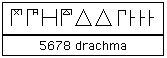5678 drachmas would be written in this way:

The form of the units would denote drachmas.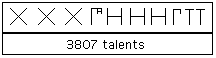3807 talents would be written as:

The units would now appear as T (T for talent). A sum of money involving both drachmas and obols would be written as: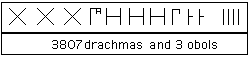3807 drachmas and 3 obols:

This acrophonic system was used for more than money. A very similar system was also used in dealing with weights and measures which is not surprising since the value of money would certainly have evolved from a system of weights. This is confirmed by the fact that the drachma was also the name of the unit of weight.

We now look at a second ancient Greek number system, the alphabetical numerals, or as it is sometimes called, the 'learned' system. As the name 'alphabetical' suggests the numerals are based on giving values to the letters of the alphabet. It is worth noting that the Greeks were one of the first to adopt a system of writing based on an alphabet. They were not the inventors of this form of writing, for the Phoenicians had such a system in place first. The Greek alphabet used to write words was taken over from the Phoenician system and was quite close to it. We will not examine the forms of the Greek letters themselves, but it is certainly worth stressing how important this form of writing was to be in advancing knowledge. It is fundamental to our ways of communicating in most countries today, although some peoples do prefer to use other forms of writing.

There are 24 letters in the classical Greek alphabet and these were used together with 3 older letters which have fallen out of use. These 27 letters are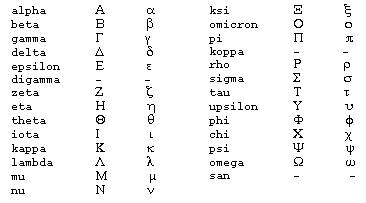Of these we have given both the upper case and lower case versions of the 24 classical letters. The letters digamma, koppa, and san are the obsolete ones. Although we have not given their symbols in the above table their symbols appear in the numeral tables below. The first nine of these letters were taken as the symbols for 1, 2, ... , 9.alphabetical 1-9.

Notice that 6 is represented by the symbol for the obsolete letter digamma.

The next nine letters were taken as the symbols for 10, 20, ... , 90.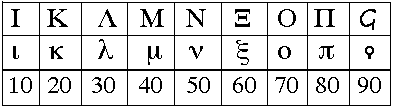alphabetical 10-90.

Notice that 90 is represented by the symbol for the obsolete letter koppa.

The remaining nine letters were taken as the symbols for 100, 200, ... , 900.alphabetical 100-900.

Notice that 900 is represented by the symbol for the obsolete letter san.

Sometimes when these letters are written to represent numbers, a bar was put over the symbol to distinguish it from the corresponding letter.

Now numbers were formed by the additive principle. For example 11, 12, ... , 19 were written:alphabetical 11-19.

Larger numbers were constructed in the same sort of way. For example here is 269.alphabetical 269.

Now this number system is compact but without modification is has the major drawback of not allowing numbers larger than 999 to be expressed. Composite symbols were created to overcome this problem. The numbers between 1000 and 9000 were formed by adding a subscript or superscript iota to the symbols for 1 to 9.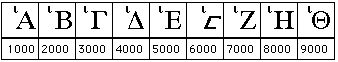First form of 1000, ..., 9000.Second form of 1000, ..., 9000.

How did the Greeks represent numbers greater than 9999? Well they based their numbers larger than this on the myriad which was 10000. The symbol M with small numerals for a number up to 9999 written above it meant that the number in small numerals was multiplied by 10000. Hence writing β above the M represented 20000: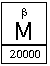The number 20000.

Similarly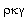written above the M represented 1230000:The number 1230000.

Of course writing a large number above the M was rather difficult so often in such cases the small numeral number was written in front of the M rather than above it. An example from Aristarchus:Aristarchus wrote the number 71755875 as:

For most purposes this number system could represent all the numbers which might arise in normal day to day life. In fact numbers as large as 71755875 would be unlikely to arise very often. On the other hand mathematicians did see the need to extend the number system and we now look at two such proposals, first one by Apollonius and then briefly one by Archimedes (although in fact historically Archimedes made his proposal nearly 50 years before Apollonius).

Although we do not have first hand knowledge of the proposal by Apollonius we do know of it through a report by Pappus. The system we have described above works with products by a myriad. The idea which Apollonius used to extend the system to larger numbers was to work with powers of the myriad. An M with an α above it represented 10000, M with β above it represented M2, namely 100000000, etc. The number to be multiplied by 10000, 10000000, etc is written after the M symbol andis written between the parts of the number, a word which is best interpreted as 'plus'. As an example here is the way that Apollonius would have written 587571750269.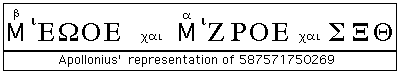Apollonius's representation of 587571750269.

Archimedes designed a similar system but rather than use 10000 = 104 as the basic number which was raised to various powers he used 100000000 = 108 raised to powers. The first octet for Archimedes consisted of numbers up to 108 while the second octet was the numbers from 108 up to 1016. Using this system Archimedes calculated that the number of grains of sand which could be fitted into the universe was of the order of the eighth octet, that is of the order of 1064.

Article by: J J O'Connor and E F Robertson

A Reference (One book/article)

 JOC/EFR © January 2001 Copyright information School of Mathematics and Statistics University of St Andrews, Scotland